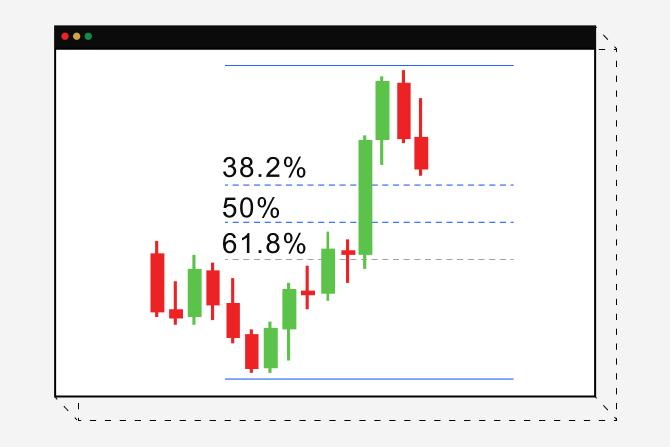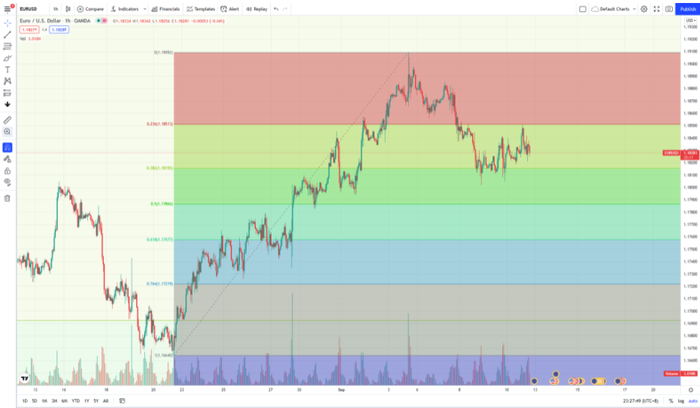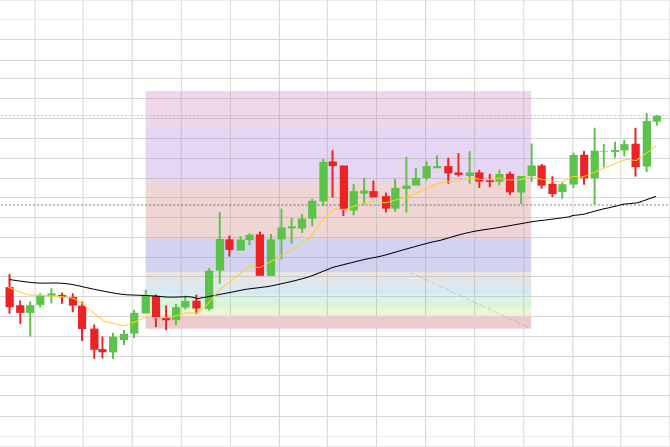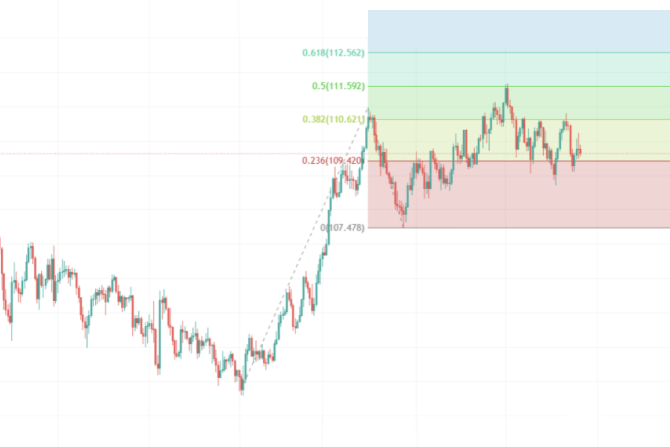CFDs are complex instruments and come with a high risk of losing money rapidly due to leverage.

76.6% of retail investor accounts lose money when trading CFDs with this provider.

You should consider whether you understand how CFDs work and whether you can afford to take the high risk of losing your money.

Choose another country or region to see content specific to your location

# How to use Fibonacci retracements and extensions

#### The Fibonacci retracement tool can help traders identify potential retracement levels in trending markets, while the Fibonacci extension identifies potential take profit zones.### What is the Fibonacci sequence?

FIbonacci is a mathematical sequence that was discovered by Leonardo Pisano Bigollo in the 11th century. The sequence is a famous group of numbers beginning with 0 and 1 and is built by adding the two numbers before it.

So, it goes like this:

1 + 1 = 2,

2 + 1 = 3,

3 + 2 = 5.

5 + 3 = 8,

5 + 8 = 13,

13 + 8 = 21,

21 + 13 = 34 and so on, where every number in the series is the previous two added together.

It’s not the sequence itself that is so important, rather it’s the quotient of the adjacent numbers. What Fibonacci found was that if you divide 8 by 5 and 13 by 8 and so on through the sequence you get the same value, that being 1.168 or dividing the numbers the opposite way, you get 0.168.

Further, if you then divide a number in the sequence by the number two further along in the sequence, say 8/21, you get 0.382. If you divide a number in the sequence by a number 3 along, say 8/34, you get 0.236. And if you divide the number 1 by the number next to it, i.e. 2, you get 0.5. These are some of the values of the Fibonacci numbers.

Interestingly, almost everything in nature has dimensional properties that reflect this ‘golden ratio’ of 1.618, which suggests this number is a fundamental function of design in nature.

You see these Fibonacci numbers everywhere in nature, from the structure of a shell to the shape of the galaxy. The argument is that if these numbers apply everywhere in nature, there’s no reason why they can’t be applied to patterns on a chart. Chartists then convert these numbers to percentages. So, 0.618 becomes 61.8%.0.5 becomes 50% and so on. Traders who use the Fibonacci tool believe that price is drawn to the different levels of the Fibonacci sequence as if by a law of nature.

### How to use the Fibonacci retracement tool

The Fib, as traders often call it, assumes the asset you are thinking of trading is in a trend, either up or down. As a tool it doesn’t provide reliable information if the market is ranging or consolidating. Once we’ve pulled up a chart that shows a strong trend, we look for major swing lows and swing highs.

A swing high will have a recent high with two lower highs on the left and right of the high itself, while a swing low is a recent low with at least two higher lows on either side of the low itself.

Now let’s take an example of how you might use the Fibonacci retracement tool on an OANDA platform.

Just below you have a chart for EUR/USD showing the base pair (EUR) beginning to break down against the Dollar. First, we select the Fib tool from the tools section on your chart. Once you’ve selected the Fib, you then click on the point of the most recent major swing high and drag the mouse down to the most recent major swing low. You’ll see the Fib maps out retracement levels between these two points; it’s these levels that represent potential areas of support and resistance and, therefore, of interest to a trader looking for entries and exits.On this hourly EUR/USD chart, we can see the start of a possible downtrend from the high. The price action slipped below the 0.236 retracement, but found some support around the 0.382 retracement before retesting the 0.236 level above and failing at the next level of resistance.

### Setting your stop loss based on Fib retracements

Many traders use Fibonacci retracements as a guide for setting their stop loss. For example, if you were to enter a buy trade at the start of an upward trend, you could place your stop loss just below the origin of the swing low. Once the reversal trend gets going, you can move your stop loss up a few pips below the next Fibonacci retracement, repeating the process until the trend begins to exhaust itself.

If you were shorting a currency pair that was in a downward trend, you would apply the opposite technique, placing your stop loss just above each successive resistance level as indicated by the Fibonacci retracement tool.

### How to use the Fibonacci Extension

This tool is slightly different from the Fibonacci retracement tool as it depends on connecting three different points on a trend in order for it to be useful, whereas the Fibonacci retracement requires only two points.

Let’s take an example. First, locate the Fibonacci extension tool - it’s in the same section as the Fib retracement tool if you’re using the TradingView platform.

Start by clicking on the bottom of the candle at the start of the impulse (or swing low in the example below), drag your mouse up to the end of that impulse where you click and draw down to the end of the retracement. The Fibonacci extension tool will spread out as you can see on the image below, giving you FIbonacci extension levels. We can then use these levels as potential profit targets.

If you look at the chart below, you can see the Fibonacci extension tool provides you with a variety of levels which could be seen to give you price points for taking profit. What you tend to find is the earlier levels are more accurate, but as the trend continues up, at least in this case, the candles start to move in a sideways fashion, closing in between the levels.### Seeking confluence for your Fibonacci extensions

You might reasonably ask yourself, how do I know which Fibonacci Extension level to set my profit target at? The following factors could be of help:

Consider the market environment. Has there been a strong breakout, for example? The stronger the trend the more likely the upper extensions could prove useful to you.

You could test for confluence by drawing in major support and resistance levels to see if your Fib extension levels coincided with your Fib-derived price point for a take profit. You could also check to see where these levels are in relation to key moving averages, such as the 50 MA.

### Limitations of the Fibonacci tools

Unlike other indicators that draw from actual price data, both Fibonacci tools work outside of any real market data. Many traders will tell you that the Fibonacci tool works only in as much that so many traders use the Fibonacci retracement tool, their decisions based on readings from the Fibonacci retracement tool could influence the movement of the markets, creating a sort of self-fulfilling prophecy.

### Key takeaways

• Fibonacci ratios are common in everyday life, and are seen in galaxy formations, snail shells and the formation of petals. This is why many traders believe these common ratios also apply to the action of price in the financial markets
• Traders use the Fibonacci retracement and extension tools to mark out levels on a chart where price can be expected to pause and reverse, and are best used in a trending market
• To use the Fibonacci retracement tool, you typically connect the swing low to the swing high (or the reverse in a bearish trend) to reveal the ratios found in the FIbonacci sequence together with a halfway point: 23.6%, 38.2%, 50%, 61.8%, and 78.6%
• The Fibonacci extension tool works by connecting the first point of an impulse wave to the first pivot to the downside (or upside in a bearish trend) and then to the swing high
• Common Fibonacci extension levels are 61.8%, 100%, 161.8%, 200%, and 261.8%
• Both retracement and extension levels can serve as areas of support and resistance but traders should consider the environment of the market, such as momentum and seasonality, before relying on them exclusively for favourable results

Difference between leveraged and other forms of financial trading.

expand_less expand_more